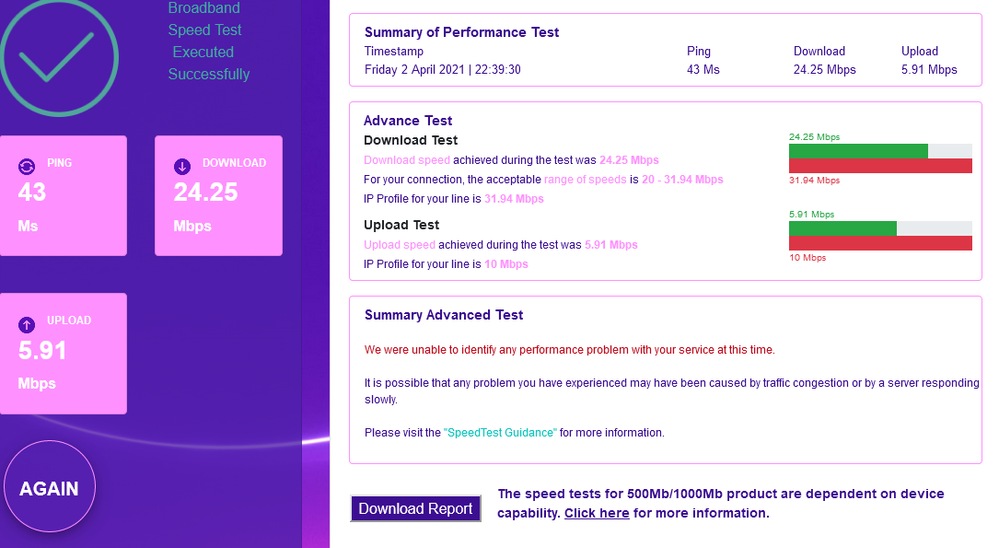cancel
Showing results for
Did you mean:

## Speed DropGrafter
Posts: 41
Thanks: 5
Fixes: 2
Registered: ‎11-11-2018

## Speed Drop

When I signed up my guaranteed speed was 34.  I have for the past year + consistently had speed test results of 35.3+ / 8.8+.

Over the past few weeks speed has dropped off and now am getting speed tests of 30 / 5.  Router log shows a dropping off of connection rates.

02:31:29, 31 Mar. Down Rate=32620Kbps, Up Rate=5691Kbps; SNR Margin Down=6.1dB, Up=6.0dB

00:31:34, 24 Mar. Down Rate=34612Kbps, Up Rate=5736Kbps; SNR Margin Down=6.1dB, Up=5.9dB

02:52:48, 16 Mar. Down Rate=36629Kbps, Up Rate=9558Kbps; SNR Margin Down=5.1dB, Up=6.0dB

00:54:13, 15 Mar. Down Rate=36691Kbps, Up Rate=8937Kbps; SNR Margin Down=5.1dB, Up=6.0dB

01:14:04, 12 Mar. Down Rate=36626Kbps, Up Rate=9494Kbps; SNR Margin Down=5.1dB, Up=5.7dB

22:33:03, 21 Feb. Down Rate=38171Kbps, Up Rate=9464Kbps; SNR Margin Down=4.6dB, Up=5.9dB

02:05:14, 14 Feb. Down Rate=38171Kbps, Up Rate=9278Kbps; SNR Margin Down=4.6dB, Up=6.0dB

20:45:21, 05 Feb. Down Rate=37069Kbps, Up Rate=9599Kbps; SNR Margin Down=5.1dB, Up=5.9dB

02:22:11, 12 Jan. Down Rate=38171Kbps, Up Rate=9987Kbps; SNR Margin Down=4.8dB, Up=5.1dB

00:37:27, 07 Jan. Down Rate=37395Kbps, Up Rate=9632Kbps; SNR Margin Down=5.2dB, Up=6.0dB

 6. Data rate: 5680 / 32995 7. Maximum data rate: 5683 / 34527 8. Noise margin: 5.8 / 6.0 9. Line attenuation: 27.3 / 16.0 10. Signal attenuation: 28.7 / 15.8Could someone have a look

TIAGrafter
Posts: 41
Thanks: 5
Fixes: 2
Registered: ‎11-11-2018

## Re: Speed Drop

Stats dropped again

00:14:27, 08 Apr. Down Rate=31008Kbps, Up Rate=5677Kbps; SNR Margin Down=6.8dB, Up=6.0dBB

 6. Data rate: 5677 / 31008 7. Maximum data rate: 5613 / 32618 8. Noise margin: 5.6 / 6.7 9. Line attenuation: 27.1 / 15.9 10. Signal attenuation: 29.1 / 15.9

So something is going on with my line